Courses

# Test: Properties of Fluids - 1

## 10 Questions MCQ Test Topicwise Question Bank for Mechanical Engineering | Test: Properties of Fluids - 1

Description
This mock test of Test: Properties of Fluids - 1 for Mechanical Engineering helps you for every Mechanical Engineering entrance exam. This contains 10 Multiple Choice Questions for Mechanical Engineering Test: Properties of Fluids - 1 (mcq) to study with solutions a complete question bank. The solved questions answers in this Test: Properties of Fluids - 1 quiz give you a good mix of easy questions and tough questions. Mechanical Engineering students definitely take this Test: Properties of Fluids - 1 exercise for a better result in the exam. You can find other Test: Properties of Fluids - 1 extra questions, long questions & short questions for Mechanical Engineering on EduRev as well by searching above.
QUESTION: 1

### What is the equivalent pressure head of mercury corresponding to 30 cm column of kerosene of relative density 0.8?

Solution: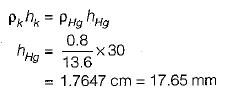QUESTION: 2

### What is dynamic viscosity in Pa-s for a liquid having relative density of 0.85 and kinematic viscosity of 2.5 centistoke?

Solution: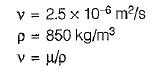or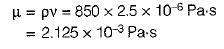QUESTION: 3

### The space between two parallel plates kept 4 mm apart is filled with an oil of dynamic viscosity 0.2 Pa-s. What is the shear stress on the lower fixed plate, if the upper one is moved with a velocity of 1.6 m/s? .

Solution: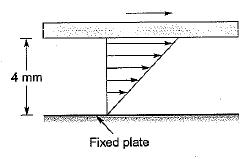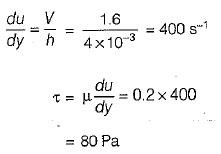QUESTION: 4

The velocity distribution in a viscous flow over a plate is given by
u = 4y - y2 for y ≤ 2m
where, u = velocity in m/s at a point distant y from the plate. If the coefficient of dynamic viscosity is 1.5 Pa-s, what is the shear stress at y= 1.2 m?

Solution: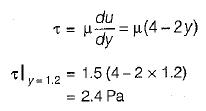QUESTION: 5

What is the pressure inside a soap bubble, over the atmospheric pressure if its diameter is 2 cm and the surface tension is 0.1 N/m?

Solution: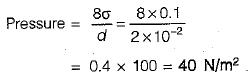QUESTION: 6

The gap between a -horizontal shaft and a concentric sleeve is filled with viscous oil. The sleeve moves with a constant velocity of 1.5 m/s when a force of 1500 N is applied parallel to the axis of the shaft. If it was required to move the sleeve at a velocity of 2 m/s, what should have been the force?

Solution: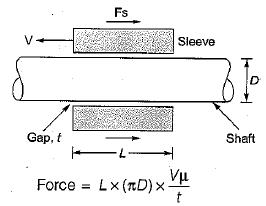for the given problem, L, D, μ and fare constant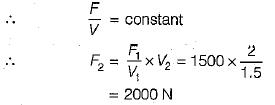QUESTION: 7

A perfect fluid is

Solution:
QUESTION: 8

The concept of continuum in fluid flow assumes that the characteristics length of the flow is

Solution:
QUESTION: 9

When a shear stress is applied to a substance, it is found to resist it by static deformation, the  substance is

Solution:
QUESTION: 10

The condition of ‘no slip’ at boundaries is applicable to

Solution: